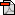Using MATLAB to improve student understanding of vector calculusUsing MATLAB to improve student understanding of vector calculus
Essay on using Matlab to teach vector calculus

File 79575 is a 80kB Acrobat (PDF)